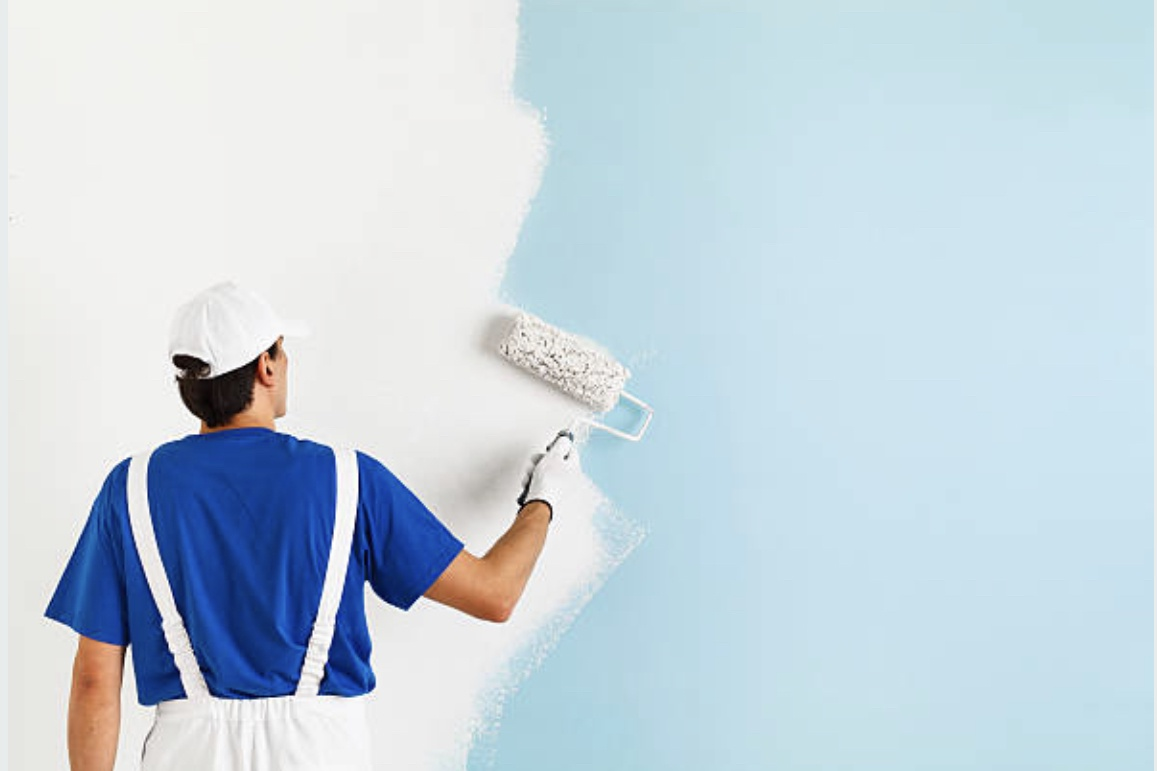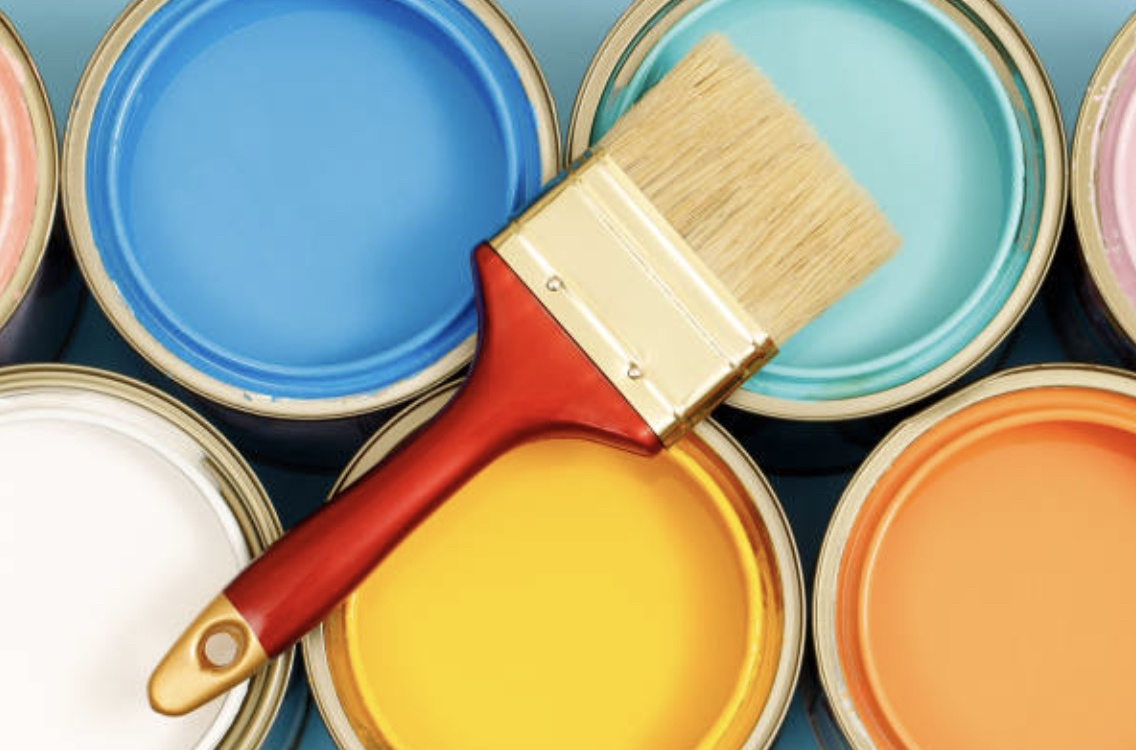Sign in# How to Calculate the Quantity of Paints Needed for a building

Painting a building is an important part of the construction process that enhances its aesthetic appeal, protects it from environmental factors, and improves its overall value. To ensure that you purchase the right amount of paint for your building project, you need to know how to calculate the quantity of paint needed. In this article, according to Wikihow, we will provide a step-by-step guide on how to calculate the quantity of paints needed for a building.Before calculating the quantity of paint needed for a building, it is important to note that the quantity of paint required depends on various factors such as the size of the building, the number of coats of paint to be applied, the type of paint used, quality of paint, and the method of application. Therefore, it is crucial to take accurate measurements and make appropriate calculations to avoid running out of paint or wasting excess paint.

Step-by-Step Guide:

Determine the surface area to be painted: Using a measuring tape, measure the length and height of each wall and ceiling to be painted. Multiply the length by the height to obtain the square footage of each wall and ceiling. Add up the square footage of all walls and ceilings to determine the total surface area to be painted.Subtract the area of doors and windows: Measure the height and width of each door and window in the room and subtract their area from the total area to be painted. For example, if the area of the windows and doors in the room is 50 square feet, subtract it from the total area of the walls.

Determine the paint coverage: The paint coverage is the amount of paint required to cover one square meter of wall surface. This information can be found on the paint can or from the paint manufacturer's website. For example, if the coverage of a particular paint is 10 square meters per liter, it means that one liter of paint will cover 10 square meters of wall surface.Calculate the total quantity of paint needed: Once you have determined the paint coverage, multiply it by the total surface area of all the walls that need to be painted. This will give you the total amount of paint required in liters. For example, if the total surface area of all the walls is 100 square meters and the paint coverage is 10 square meters per liter, then you will need 10 liters of paint.

Account for multiple coats: If you are planning to apply more than one coat of paint, you need to account for this in your calculations. Multiply the total surface area by the number of coats you plan to apply. For example, if you plan to apply two coats of paint, you will need 20 liters of paint based on the above example.

Adjust for waste: It is important to factor in some waste when calculating the amount of paint needed for a building. This is because some paint will be lost due to spillage, overspray, and uneven application. A good rule of thumb is to add an extra 10% to 20% to the total amount of paint calculated.

Working example:

Here's an example to illustrate the calculation:

Suppose you have a building with four walls, each measuring 10 meters in length and 3 meters in height. The total area of the walls is:

10m x 3m = 30m² (for each wall)

30m² x 4 = 120m² (for all four walls)

Suppose there are also four windows, each measuring 1.5 meters in height and 1 meter in width, and one door measuring 2.2 meters in height and 1 meter in width. The total area of the openings is:

1.5m x 1m = 1.5m² (for each window)

1.5m² x 4 = 6m² (for all four windows)

2.2m x 1m = 2.2m² (for the door)

6m² + 2.2m² = 8.2m² (for all openings)

The net area to be painted is:

120m² - 8.2m² = 111.8m²

Suppose the paint you plan to use has a coverage rate of 10m²/L. The quantity of paint needed is:

111.8m² ÷ 10m²/L = 11.18L

Round up to the nearest whole number to get the final quantity of paint needed. 11.18L rounded up to the nearest whole number = 12L

Therefore, you will need 12 liters of paint to cover the walls of this building, excluding the windows and doors.

Note: If you plan to apply two coats of paint, you will need (12x2=24) liters of paint based on the above example.

Account for extra paint needed. To be on the safe side, we'll add 10% to the total quantity of paint needed.

Extra paint needed = 12 x 0.10

Extra paint needed = 1.2liters

Total paint needed = 12 + 1.2

Total paint needed = 13.2 liters

Note: In total, you will need 13-14 liters of paints.By following these steps, you can estimate the amount of paint needed for your building project, which can help you plan your budget and ensure that you have enough paint to complete the job.

Content created and supplied by: Dr.Love (via Opera News )